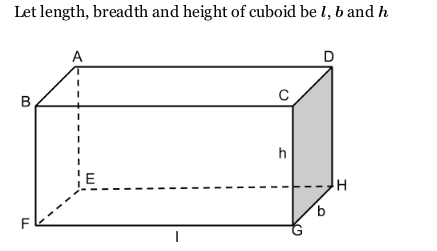# What is the lateral surface area of a cuboid?

Solution:

The lateral surface area of a cuboid is the sum of the areas of its 4 faces (excluding the top and bottom face)A cuboid has six faces.

Area of BFGC and AEHD {each} = $h \times l$

Area of ABFE and CGHD {each} = $b \times h$

Area of ABCD and EFGH (each )=$l \times b$

Therefore, area of all faces =$2(h \times l)+2(b \times h)+2(l \times b)$

=$2(h\times l+b \times h+l \times b)$

=$2(l\times b+b \times h+h \times l)$

It is called total surface area of a cuboid.

Therefore,

Lateral surface area of a cuboid $=2(l\times b+b \times h+h \times l)-2(l \times b)$

$=2(b \times h+h \times l)$

Lateral surface area of a cuboid of length $l$, breadth $b$ and height $h$ is $2(b \times h+h \times l)$.